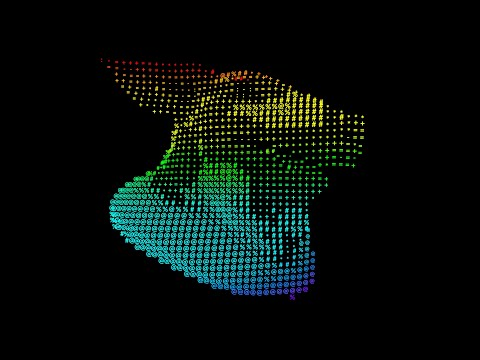# Turning images into ASCII art

calendar_today

24.06.2022

label

VEX, Rendering

mouse

Houdini 19.0

### 1 Console versionAfter applying an image to a grid of rows using attribute from map, we loop through each line point by point to convert the luminance to a set of characters with increasing visual density:
`. : - = + * # % @`. Then we print it to the console using `\n` for line breaks and optionally disable the commenting for `reverse(chars)` to invert the image.

``````string chars[] = array(' ','.',':','-','=','+','*','#','%','@');
//chars = reverse(chars);
int prims[] = expandprimgroup(0, '*');
foreach(int pr; prims){
int pts[] = primpoints(0, pr);
foreach(int pt; pts){
float a = point(0, 'a', pt);
int index = int(a * 9);
printf('%g', chars[index]);
}
printf('\n');
}
printf('\n');``````

### 2 SOP font versionTransforming image brightness into characters by using the variant attribute on the copy to points-node.

Centering letters on top of each other before copying is done by index with VEX:

``````int index = prim(0, 'textindex', i@primnum);
string grp = '@textindex==' + itoa(index);
vector center = getbbox_center(0, grp);

v@P.x -= center.x;``````

### 3 Raycasting meshesRaycasting mesh attributes and adding depth blur into a 2D volume.

Posterized colors values `val` are turned into text indices by counting the number of unique indices `nuniqueval` from the font SOP on second input.

``````string a = chs('attribute');
float val = point(0, a, i@ptnum);
int num = nuniqueval(1, 'prim', 'textindex');
i@textindex = int(val * (num - 1));``````

The blur mask is created based on the remapped distance gathered from the Ray node.